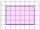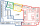# Mixed fractions calculator

The calculator performs basic and advanced operations with mixed numbers, fractions, integers, decimals. Mixed fractions are also called mixed numbers. A mixed fraction is a whole number and a proper fraction combined, i.e. one and three-quarters. The calculator evaluates the expression or solves the equation with step-by-step calculation progress information. Solve problems with two or more mixed numbers fractions in one expression.

The calculator performs basic and advanced operations with mixed numbers, fractions, integers, decimals. Mixed fractions are also called mixed numbers. A mixed fraction is a whole number and a proper fraction combined, i.e. one and three-quarters. The calculator evaluates the expression or solves the equation with step-by-step calculation progress information. Solve problems with two or more mixed numbers fractions in one expression.

## Result:

### 3/4 + 2/3 = 17/12 = 1 5/12 ≅ 1.4166667

Spelled result in words is one and five twelfths (or seventeen twelfths).

### Calculation steps

1. Add: 3/4 + 2/3 = 3 · 3/4 · 3 + 2 · 4/3 · 4 = 9/12 + 8/12 = 9 + 8/12 = 17/12
For adding, subtracting, and comparing fractions, it is suitable to adjust both fractions to a common (equal, identical) denominator. The common denominator you can calculate as the least common multiple of both denominators - LCM(4, 3) = 12. In practice, it is enough to find the common denominator (not necessarily the lowest) by multiplying the denominators: 4 × 3 = 12. In the next intermediate step, the fraction result cannot be further simplified by canceling.
In words - three quarters plus two thirds = seventeen twelfths.

#### Examples:

sum of two mixed numbers: 1 3/4 + 2 3/8
addition of three mixed numbers: 1 3/8 + 6 11/13 + 5 7/8
addition of two mixed numbers: 2 1/2 + 4 2/3
subtracting two mixed numbers: 7 1/2 - 5 3/4
multiplication of mixed numbers: 3 3/4 * 2 2/5
comparing mixed numbers: 3 1/4 2 1/3
changing improper fraction to mixed number: 9/4
What is 3/4 as a mixed number: 3/4
subtracting mixed number and fraction: 1 3/5 - 5/6
sum mixed number and an improper fraction: 1 3/5 + 11/5

## Mixed fractions in word problems:

• Mixed2improperWrite the mixed number as an improper fraction. 166 2/33 3/4 + 2 3/5 + 5 1/2 Show your solution.
• Mixed divided with fracThere are 2 2/3 pizzas. How many people are sharing when each has 2/3 of pizza?2 and 1/8th plus 1 and 1/3rd =
• GavinGavin had 3 gallons of orange juice ready to serve at the breakfast party. His family drank 2 ⅛ gallons. How much is left? Show your work with a numberline, area model or grid.Add two mixed fractions: 2 4/6 + 1 3/6
• Equation with mixed fractions2 3/5 of 1430+? = 1900. How to do this problem
• Sum of mixedThe sum of two mixed numbers is 15½. One of the number is 5 ¾, what is the other?
• An electricianAn electrician needs 1 1/3 rolls of electrical wire to wire each room in a house. How many rooms can he wire with 6 2/3 rolls of wire?
• Donuts 3You pick up donuts for a party. You have 3 and 4/12 boxes of donuts. Roy picks up donuts too. He has 2 and 9/12 boxes of donuts. How many boxes of donuts do you have in all?
• Cups of punchCyka made 6 19/20 cups of punch punch at two different types of juice in it. If the punch had 4 1/5 cups of one type of juice how many cups of the other type of juice did it have?
• Apples 14Maya bought 18 apples. She used 50% of the apples to make apple pies and 1/3 of the apples was mixed with other fruits to make salad. How many apples were left?
• Jack plantedJack planted 1 1/4 rows of lettuce in the family garden, Rose planted 1 1/2 rows of broccoli and Calvin planted 4 5/12 rows of beans. How many rows were planted in the garden?
• Bike pathBill was riding his bike on a bike path that was 5 1/4 miles long. He rode his bike awhile and stopped for s drink or water. Then he rode 2 7/10 miles and was at the end of the path. How far had Bill ridden before he stopped for water?Add this two mixed numbers: 1 5/6 + 2 2/11=What is the difference between 4 2/3 and 3 1/6?The first team member in a 926-person relay race must run 2 1/4 laps, the second team member must run 1 1/2 laps, and the third team member must run 3 1/4 laps. How many laps in all must each team run?